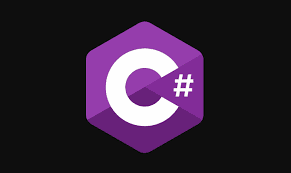In this article we will discuss what is the Static and Non-Static Members in C# with some examples. We will cover the below points in this article.
• What are static and non-static members in C#?
• When do we need to use static and non-static members in C#?
• Static and Non-static variables in C#.
• What is the scope of Non-Static variables in C#?
What are static and non-static members in C#?

The member of a class is divided into two categories
• Static members   - The members of a class which does not require an instance for initialization or execution are known as the static member.
• Non-static members - The members which require an instance of a class for both initialization and execution are known as non-static members.
When do we need to use static and non-static members in C# ?

Whenever we declare a variable by using the static modifier or when we declare a variable inside of any static block then those variables are considered as static variables whereas the rest of the other are considered as non-static variables.

If you want a variable to have the same value throughout all instances of a class then you need to declare that variable as a static variable. So, the static variables are going to hold the application level data which is going to be the same for all the objects.

The static variable gets initialized immediately once the execution of the class starts whereas the non-static variables are initialized only after creating the object of the class and that is too for each time the object of the class is created.

A static variable gets initialized only once during the life cycle of a class whereas a non-static variable gets initialized either 0 or n number of times, depending on the number of objects created for that class.

If you want to access the static members of a class, then you need to access them using the class name whereas you need an instance of a class to access the non-static members.

What is the scope of Non-Static variables in C# ?

The Non Static variables are created when the object is created and are destroyed when the object is destroyed. The object is destroyed when its reference variable is destroyed or initialized with null. So we can say that the scope of the object is the scope of its referenced variables.

Static and Non-Static methods in C#

If we declare a method using the static modifier then it is called as a static method else it is a non-static method. You cannot consume the non-static members directly within a static method. If you want to consume any non-static members with a static method then you need to create an object that and then through the object you can access the non-static members. On the other hand, you can directly consume the static members within a non-static method without any restriction.

Rules to follow while working with static and non-static members in c#:

Non-static to static: Can be consumed only by using the object of that class.
Static to static: Can be consumed directly or by using the class name.
Static to non-static: Can be consumed directly or by using the class name.
Non-static to non-static: Can be consumed directly or by using the “this” keyword.

### You May Also Like...

Summary
In this tutorial we discussed static and non-static members in C#. If have any question related to this topic then give your feedback.